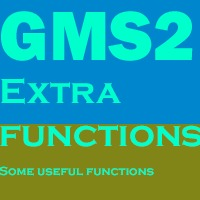# Extra Functions

#### Black Smite

You must be logged in to obtain assets

Contact Publisher

### Description

A few extra functions that are useful,a few of them are-

-percent_chance(chance) eg. if(percent_chance(50)); { //Do something } there is a 50% chance this will return true.

-approach(variable, target, increment/decrement) eg. Imagine that x = 10 and y = 20 Step Event: x = approach(x,y,1); This will add 1 to x each step until it is equal to y. or y = approach(y,x,1); this will subtract 1 from y each step until it is equal to x

-rotate_towards_point(currentangle,targetangle,rotationspeed) eg. var mangle = point_direction(x,y,mouse_x,mouse_y); image_angle = rotate_towards_point(image_angle, mangle,5); This will smoothly rotate the image to the mouse with a rotation speed of 5.

-move_flee(x,y) The opposite of move_towards_point(x,y) moves away from the given x & y

+More!